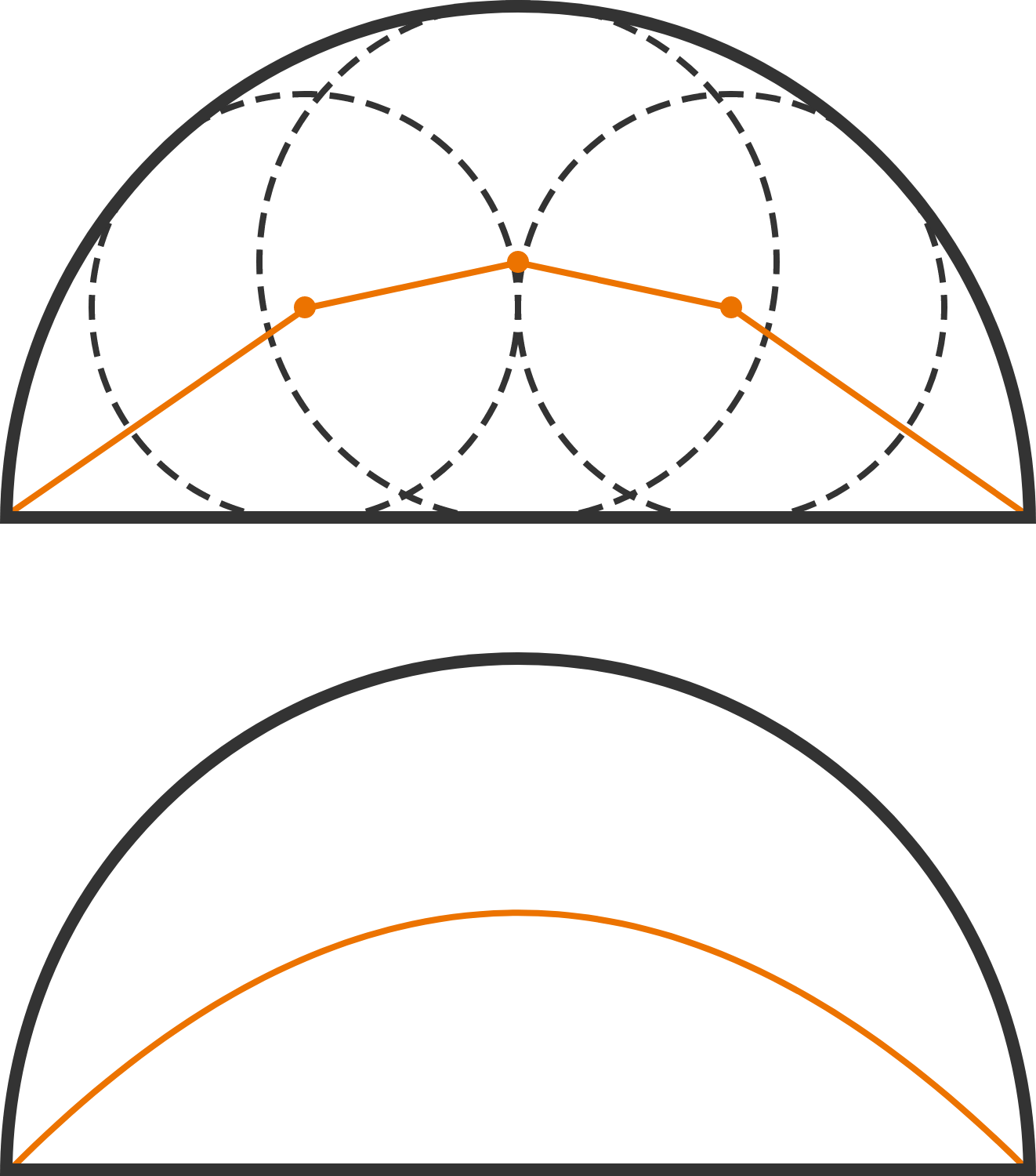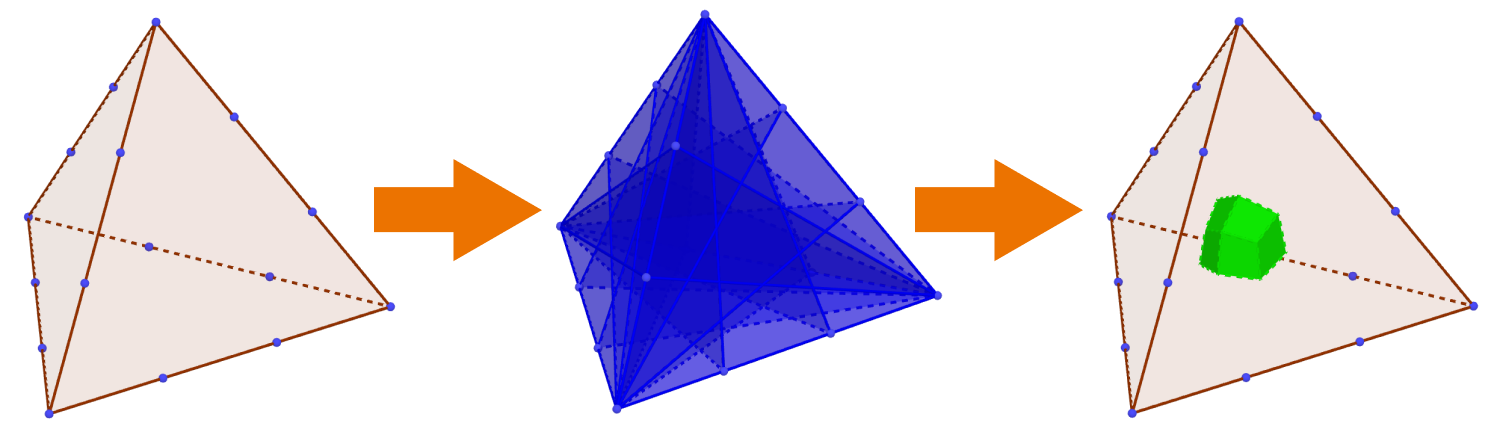# Problems of the Week

Contribute a problem

You have 2016 sticks of the same length in a box. You pick a stick at random, break it into two equal halves, and put them back in for a total of 2017 sticks. You repeat this process of random picking and breaking indefinitely.

What is the maximum value of $x$ such that, at any time during this process, you are guaranteed to have at least $x$ sticks of the same length?

We have a partition of 2018. If the maximum value of the product of the numbers in the partition can be expressed as $a \times b^c,$ where $a$ and $b$ are primes and $c$ is an integer, then what is $a+b+c?$

The first diagram shows 3 circles inscribed in a semicircle. The orange line shows their centers connected together with the endpoints of the diameter of the semicircle.

If we inscribe infinitely many circles, not just 3, the resulting orange curve is part of what kind of curve?This problem can be viewed as the 3D analog of Marion's theorem.

Imagine that each edge of a tetrahedron is trisected. Then, through each of these 12 points and its two opposite vertices, a plane is constructed for a total of 12 planes.Now, let $V$ denote the volume of the tetrahedron, and $V_M$ the volume of the 3D figure carved out by the 12 planes inside the tetrahedron. If $V_M=\frac{A}{B}V,$ where $A$ and $B$ are coprime positive integers, find $A+B.$

The 3D figure in question is shown below:Let $S_n=\dfrac{1}{1^n}+\dfrac{1+\frac{1}{2}}{2^n}+\dfrac{1+\frac{1}{2}+\frac{1}{3}}{3^n}+\cdots$.

Then, for positive even numbers $m$, there is a beautiful relationship between $S_{m}$ and the Riemann zeta function $\zeta(\cdot)$: $\begin{array} { l r c } S_2 &=& 2\zeta(3) \\ S_4 &=& {3\zeta(5)} \quad {-\zeta(2)\zeta(3)} \\ S_6 &=& {4\zeta(7)}\quad {-\zeta(2)\zeta(5)} \quad {-\zeta(3)\zeta(4)} \\ S_8 &=& {5\zeta(9)}\quad {-\zeta(2)\zeta(7)} \quad {-\zeta(3)\zeta(6)} \quad {-\zeta(4)\zeta(5)} \\ & \vdots & \\ S_{m} &=& \displaystyle \frac{m+2}2 \zeta(m+1) - \sum_{k=2}^{\frac m2} \zeta(k) \zeta(m-k). \end{array}$ However, there is also a relationship between positive odd numbers $m$ and the Riemann zeta function. Find this relationship and submit your answer as $\dfrac{\pi^4}{S_3}.$

Bonus: Prove the pattern shown above.

×